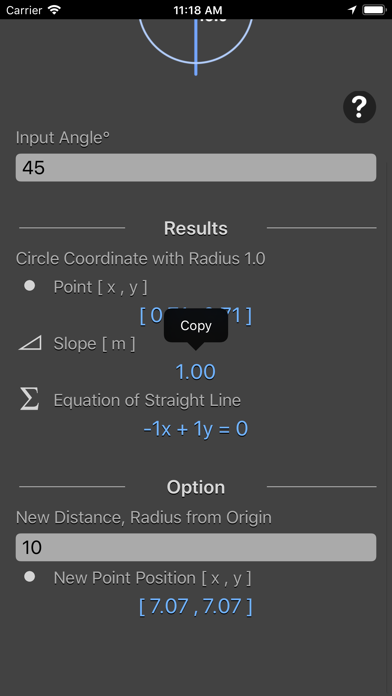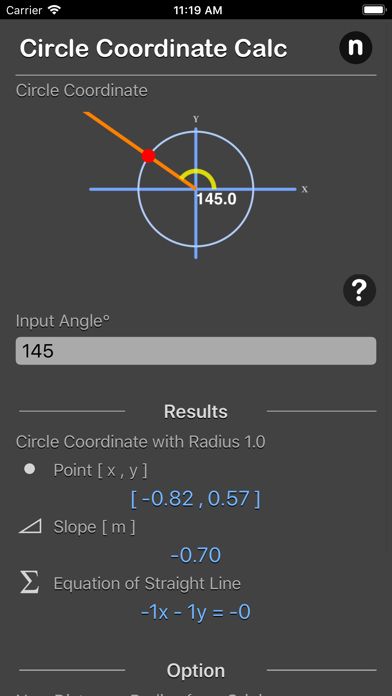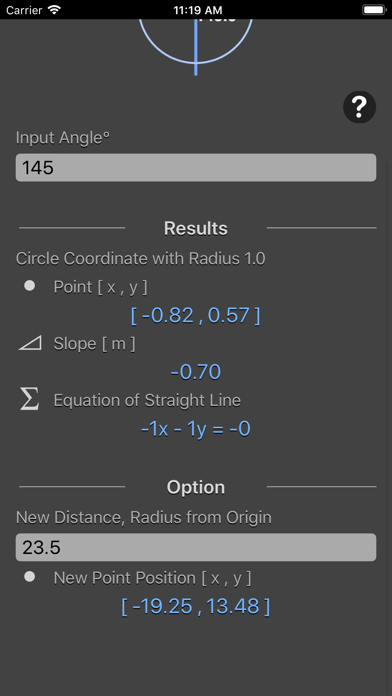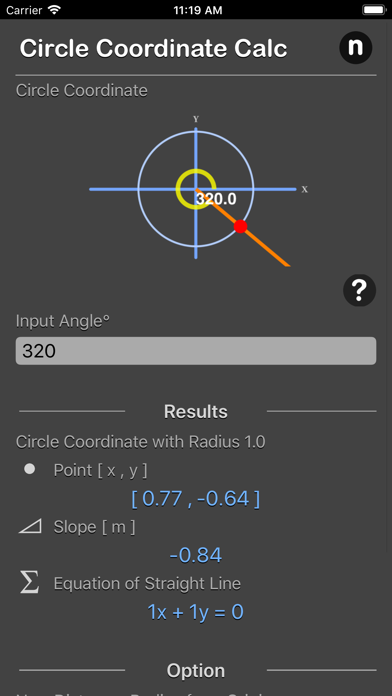## Circle Coordinate Calculator - Lumos Educational App StoreNA1
Price -\$2.99
\$2.99

#### DESCRIPTION:

Unit Circle Coordinate Calculator calculates the sine, cosine (x , y) values of any given angle. Features: - Calculates the sine, cosine (x , y) values of any given angle. - Calculates the slope (m) for the line - Output the formula for the line - Output the x coordinate and y coordinate by given new distance from origin - Result are instant and copy-able Unit circle is a circle which is centered at origin and which has the radius of 1 unit. It is represented by the equation: x^2 + y^2 = 1. The values of x and y are given by trigonometric functions as: x = sin θ y = cos θ So the value of

#### OVERVIEW:

Circle Coordinate Calculator is a free educational mobile app By Nitrio.It helps students practice the following standards .

This page not only allows students and teachers download Circle Coordinate Calculator but also find engaging Sample Questions, Videos, Pins, Worksheets, Books related to the following topics.

Developer: Nitrio

Developer URL: http://www.nitrio.com

Software Version: 1.0

Category: Utilities

Release Date: 2018-05-06T17:50:35Z#### Are you the Developer?EdSearch WebSearch